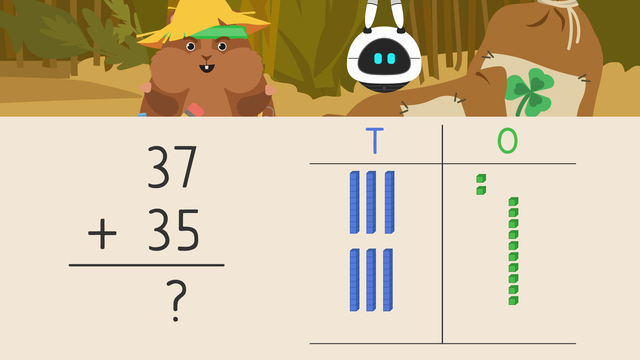# Using Place Value to Add Two Digit Numbers (Regrouping)

Content Using Place Value to Add Two Digit Numbers (Regrouping)Rating

Ø 4.5 / 6 ratings
The authorsTeam Digital
Using Place Value to Add Two Digit Numbers (Regrouping)
CCSS.MATH.CONTENT.2.NBT.B.5

## 2 Digit Place Value Addition

In this text, we’ll have a detailed look at how you solve addition problems in math using place value. But first, let’s repeat what place value is:

What is place value and how can it help when adding when you need to regroup? Place value is the value of each digit depending on where it is in the number, and is a great strategy to help when adding.

When adding using place value with regrouping, start by representing the addends using base ten blocks and rods. Remember that addends are the numbers we are adding together.

Next, add the ones blocks from each addend together. Here you can see if your ones add up to make a ten. If they do you must move it over to the tens place. This is called regrouping.

Once you add the ones blocks together, you can add the tens rods together.

## Place Value Addition with Regrouping – Practice

If you are adding thirty-five red mushrooms and seventeen brown mushrooms together, how many will you have in total?

You can use place value with regrouping to add!You will have fifty-two mushrooms all together.

## 2 Digit Place Value Addition with Regrouping – Summary

Remember, when adding using place value, regrouping works like this:

Step # What to do
1 Represent the addends with base ten blocks